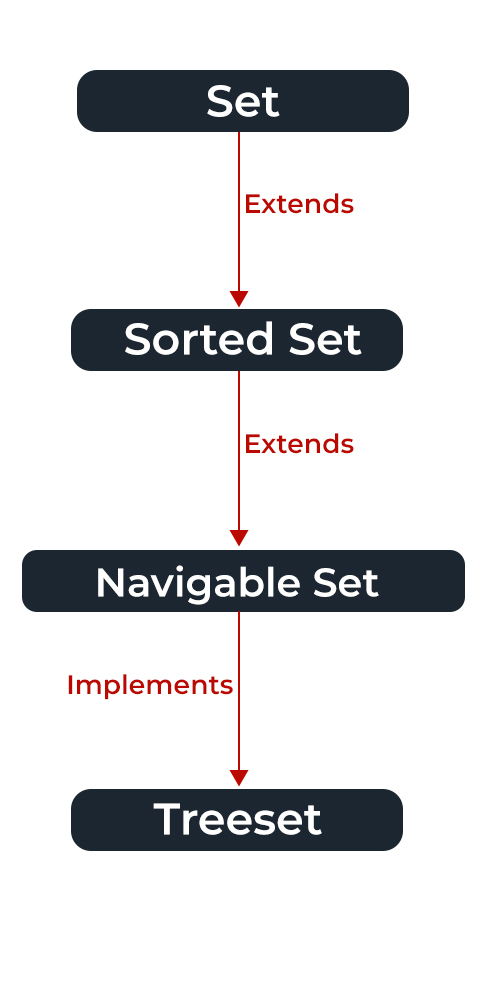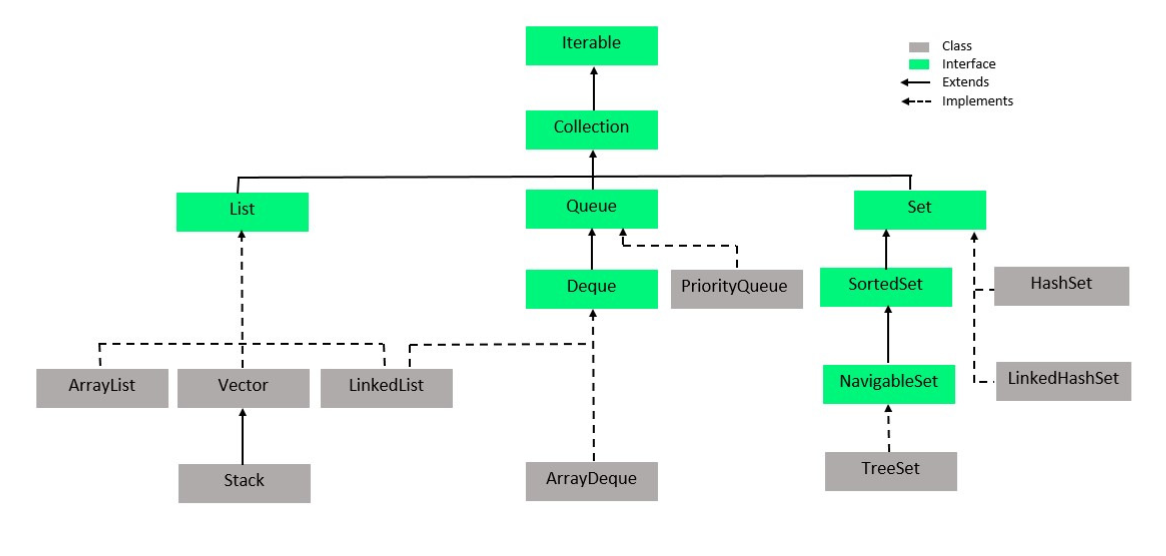# Set in Java

• Difficulty Level : Easy
• Last Updated : 26 Jul, 2022

The set interface is present in java.util package and extends the Collection interface is an unordered collection of objects in which duplicate values cannot be stored. It is an interface that implements the mathematical set. This interface contains the methods inherited from the Collection interface and adds a feature that restricts the insertion of the duplicate elements. There are two interfaces that extend the set implementation namely SortedSet and NavigableSet.In the above image, the navigable set extends the sorted set interface. Since a set doesn’t retain the insertion order, the navigable set interface provides the implementation to navigate through the Set. The class which implements the navigable set is a TreeSet which is an implementation of a self-balancing tree. Therefore, this interface provides us with a way to navigate through this tree.

Declaration: The Set interface is declared as:

`public interface Set extends Collection `

Creating Set Objects

Since Set is an interface, objects cannot be created of the typeset. We always need a class that extends this list in order to create an object. And also, after the introduction of Generics in Java 1.5, it is possible to restrict the type of object that can be stored in the Set. This type-safe set can be defined as:

```// Obj is the type of the object to be stored in Set
Set<Obj> set = new HashSet<Obj> (); ```

Let us discuss methods present in the Set interface provided below in a tabular format below as follows:

Illustration: Sample Program to Illustrate Set interface

## Java

 `// Java program Illustrating Set Interface`` ` `// Importing utility classes``import` `java.util.*;`` ` `// Main class ``public` `class` `GFG {``   ` `    ``// Main driver method``    ``public` `static` `void` `main(String[] args)``    ``{``        ``// Demonstrating Set using HashSet``        ``// Declaring object of type String ``        ``Set hash_Set = ``new` `HashSet();`` ` `        ``// Adding elements to the Set``        ``// using add() method``        ``hash_Set.add(``"Geeks"``);``        ``hash_Set.add(``"For"``);``        ``hash_Set.add(``"Geeks"``);``        ``hash_Set.add(``"Example"``);``        ``hash_Set.add(``"Set"``);`` ` `        ``// Printing elements of HashSet object``        ``System.out.println(hash_Set);``    ``}``}`

Output

`[Set, Example, Geeks, For]`

Operations on the Set Interface

The set interface allows the users to perform the basic mathematical operation on the set. Let’s take two arrays to understand these basic operations. Let set1 = [1, 3, 2, 4, 8, 9, 0] and set2 = [1, 3, 7, 5, 4, 0, 7, 5]. Then the possible operations on the sets are:

1. Intersection: This operation returns all the common elements from the given two sets. For the above two sets, the intersection would be:

`Intersection = [0, 1, 3, 4] `

2. Union: This operation adds all the elements in one set with the other. For the above two sets, the union would be:

`Union = [0, 1, 2, 3, 4, 5, 7, 8, 9] `

3. Difference: This operation removes all the values present in one set from the other set. For the above two sets, the difference would be:

`Difference = [2, 8, 9]`

Now let us implement the following operations as defined above as follows:

Example:## Java

 `// Java Program Demonstrating Operations on the Set``// such as Union, Intersection and Difference operations `` ` `// Importing all utility classes``import` `java.util.*;`` ` `// Main class ``public` `class` `SetExample {``   ` `    ``// Main driver method ``    ``public` `static` `void` `main(String args[])``    ``{``        ``// Creating an object of Set class ``        ``// Declaring object of Integer type ``        ``Set a = ``new` `HashSet();``       ` `        ``// Adding all elements to List ``        ``a.addAll(Arrays.asList(``            ``new` `Integer[] { ``1``, ``3``, ``2``, ``4``, ``8``, ``9``, ``0` `}));``       ` `      ``// Again declaring object of Set class``      ``// with reference to HashSet``        ``Set b = ``new` `HashSet();``         ` `      ``b.addAll(Arrays.asList(``            ``new` `Integer[] { ``1``, ``3``, ``7``, ``5``, ``4``, ``0``, ``7``, ``5` `}));`` ` `         ` `        ``// To find union``        ``Set union = ``new` `HashSet(a);``        ``union.addAll(b);``        ``System.out.print(``"Union of the two Set"``);``        ``System.out.println(union);`` ` `        ``// To find intersection``        ``Set intersection = ``new` `HashSet(a);``        ``intersection.retainAll(b);``        ``System.out.print(``"Intersection of the two Set"``);``        ``System.out.println(intersection);`` ` `        ``// To find the symmetric difference``        ``Set difference = ``new` `HashSet(a);``        ``difference.removeAll(b);``        ``System.out.print(``"Difference of the two Set"``);``        ``System.out.println(difference);``    ``}``}`

Output

```Union of the two Set[0, 1, 2, 3, 4, 5, 7, 8, 9]
Intersection of the two Set[0, 1, 3, 4]
Difference of the two Set[2, 8, 9]```

## Performing Various Operations on SortedSet

After the introduction of Generics in Java 1.5, it is possible to restrict the type of object that can be stored in the Set. Since Set is an interface, it can be used only with a class that implements this interface. HashSet is one of the widely used classes which implements the Set interface. Now, let’s see how to perform a few frequently used operations on the HashSet. We are going to perform the following operations as follows:

2. Accessing elements
3. Removing elements
4. Iterating elements
5. Iterating through Set

Now let us discuss these operations individually as follows:

In order to add an element to the Set, we can use the add() method. However, the insertion order is not retained in the Set. Internally, for every element, a hash is generated and the values are stored with respect to the generated hash. the values are compared and sorted in ascending order. We need to keep a note that duplicate elements are not allowed and all the duplicate elements are ignored. And also, Null values are accepted by the Set.

Example

## Java

 `// Java Program Demonstrating Working of Set by``// Adding elements using add() method `` ` `// Importing all utility classes``import` `java.util.*;`` ` `// Main class``class` `GFG {`` ` `    ``// Main driver method``    ``public` `static` `void` `main(String[] args)``    ``{``        ``// Creating an object of Set and ``        ``// declaring object of type String``        ``Set hs = ``new` `HashSet();`` ` `        ``// Adding elements to above object``        ``// using add() method``        ``hs.add(``"B"``);``        ``hs.add(``"B"``);``        ``hs.add(``"C"``);``        ``hs.add(``"A"``);`` ` `        ``// Printing the elements inside the Set object``        ``System.out.println(hs);``    ``}``}`

Output

`[A, B, C]`

Operation 2: Accessing the Elements

After adding the elements, if we wish to access the elements, we can use inbuilt methods like contains().

Example

## Java

 `// Java code to demonstrate Working of Set by``// Accessing the Elements of the Set object`` ` `// Importing all utility classes``import` `java.util.*;`` ` `// Main class``class` `GFG {`` ` `    ``// Main driver method``    ``public` `static` `void` `main(String[] args)``    ``{``        ``// Creating an object of Set and ``        ``// declaring object of type String``        ``Set hs = ``new` `HashSet();`` ` `        ``// Elements are added using add() method``        ``// Later onwards we will show accessing the same`` ` `        ``// Custom input elements``        ``hs.add(``"A"``);``        ``hs.add(``"B"``);``        ``hs.add(``"C"``);``        ``hs.add(``"A"``);`` ` `        ``// Print the Set object elements``        ``System.out.println(``"Set is "` `+ hs);`` ` `        ``// Declaring a string``        ``String check = ``"D"``;`` ` `        ``// Check if the above string exists in``        ``// the SortedSet or not``        ``// using contains() method``        ``System.out.println(``"Contains "` `+ check + ``" "``                           ``+ hs.contains(check));``    ``}``}`

Output

```Set is [A, B, C]
Contains D false```

Operation 3: Removing the Values

The values can be removed from the Set using the remove() method.

Example

## Java

 `// Java Program Demonstrating Working of Set by``// Removing Element/s from the Set`` ` `// Importing all utility classes``import` `java.util.*;`` ` `// Main class``class` `GFG {`` ` `    ``// Main driver method``    ``public` `static` `void` `main(String[] args)``    ``{``        ``// Declaring object of Set of type String``        ``Set hs = ``new` `HashSet();`` ` `        ``// Elements are added``        ``// using add() method`` ` `        ``// Custom input elements``        ``hs.add(``"A"``);``        ``hs.add(``"B"``);``        ``hs.add(``"C"``);``        ``hs.add(``"B"``);``        ``hs.add(``"D"``);``        ``hs.add(``"E"``);`` ` `        ``// Printing initial Set elements``        ``System.out.println(``"Initial HashSet "` `+ hs);`` ` `        ``// Removing custom element``        ``// using remove() method``        ``hs.remove(``"B"``);`` ` `        ``// Printing Set elements after removing an element``        ``// and printing updated Set elements``        ``System.out.println(``"After removing element "` `+ hs);``    ``}``}`

Output

```Initial HashSet [A, B, C, D, E]
After removing element [A, C, D, E]```

Operation 4: Iterating through the Set

There are various ways to iterate through the Set. The most famous one is to use the enhanced for loop.

Example

## Java

 `// Java Program to Demonstrate Working of Set by ``// Iterating through the Elements `` ` `// Importing utility classes ``import` `java.util.*;`` ` `// Main class ``class` `GFG {`` ` `    ``// Main driver method``    ``public` `static` `void` `main(String[] args)``    ``{``        ``// Creating object of Set and declaring String type``        ``Set hs = ``new` `HashSet();`` ` `        ``// Adding elements to Set  ``        ``// using add() method`` ` `        ``// Custom input elements ``        ``hs.add(``"A"``);``        ``hs.add(``"B"``);``        ``hs.add(``"C"``);``        ``hs.add(``"B"``);``        ``hs.add(``"D"``);``        ``hs.add(``"E"``);`` ` `        ``// Iterating through the Set``        ``// via for-each loop ``        ``for` `(String value : hs)`` ` `            ``// Printing all the values inside the object ``            ``System.out.print(value + ``", "``);``         ` `        ``System.out.println();``    ``}``}`

Output

`A, B, C, D, E, `

Classes that implement the Set interface in Java Collections can be easily perceived from the image below as follows and are listed as follows:

• HashSet
• EnumSet
• TreeSetClass 1: HashSet

HashSet class which is implemented in the collection framework is an inherent implementation of the hash table data structure. The objects that we insert into the HashSet do not guarantee to be inserted in the same order. The objects are inserted based on their hashcode. This class also allows the insertion of NULL elements. Let’s see how to create a set object using this class.

Example

## Java

 `// Java program Demonstrating Creation of Set object``// Using the Hashset class`` ` `// Importing utility classes``import` `java.util.*;`` ` `// Main class``class` `GFG {`` ` `    ``// Main driver method``    ``public` `static` `void` `main(String[] args)``    ``{``        ``// Creating object of Set of type String``        ``Set h = ``new` `HashSet();`` ` `        ``// Adding elements into the HashSet``        ``// using add() method`` ` `        ``// Custom input elements``        ``h.add(``"India"``);``        ``h.add(``"Australia"``);``        ``h.add(``"South Africa"``);`` ` `        ``// Adding the duplicate element``        ``h.add(``"India"``);`` ` `        ``// Displaying the HashSet``        ``System.out.println(h);`` ` `        ``// Removing items from HashSet``        ``// using remove() method``        ``h.remove(``"Australia"``);``        ``System.out.println(``"Set after removing "``                           ``+ ``"Australia:"` `+ h);`` ` `        ``// Iterating over hash set items``        ``System.out.println(``"Iterating over set:"``);`` ` `        ``// Iterating through iterators``        ``Iterator i = h.iterator();`` ` `        ``// It holds true till there is a single element``        ``// remaining in the object``        ``while` `(i.hasNext())`` ` `            ``System.out.println(i.next());``    ``}``}`

Output

```[South Africa, Australia, India]
Set after removing Australia:[South Africa, India]
Iterating over set:
South Africa
India```

Class 2: EnumSet

EnumSet class which is implemented in the collections framework is one of the specialized implementations of the Set interface for use with the enumeration type. It is a high-performance set implementation, much faster than HashSet. All of the elements in an enum set must come from a single enumeration type that is specified when the set is created either explicitly or implicitly. Let’s see how to create a set object using this class.

Example

## Java

 `// Java program to demonstrate the``// creation of the set object``// using the EnumSet class``import` `java.util.*;`` ` `enum` `Gfg { CODE, LEARN, CONTRIBUTE, QUIZ, MCQ }``;`` ` `public` `class` `GFG {`` ` `    ``public` `static` `void` `main(String[] args)``    ``{``        ``// Creating a set``        ``Set set1;`` ` `        ``// Adding the elements``        ``set1 = EnumSet.of(Gfg.QUIZ, Gfg.CONTRIBUTE,``                          ``Gfg.LEARN, Gfg.CODE);`` ` `        ``System.out.println(``"Set 1: "` `+ set1);``    ``}``}`

Output

`Set 1: [CODE, LEARN, CONTRIBUTE, QUIZ]`

LinkedHashSet class which is implemented in the collections framework is an ordered version of HashSet that maintains a doubly-linked List across all elements. When the iteration order is needed to be maintained this class is used. When iterating through a HashSet the order is unpredictable, while a LinkedHashSet lets us iterate through the elements in the order in which they were inserted. Let’s see how to create a set object using this class.

Example

## Java

 `// Java program to demonstrate the``// creation of Set object using``// the LinkedHashset class``import` `java.util.*;`` ` `class` `GFG {`` ` `    ``public` `static` `void` `main(String[] args)``    ``{``        ``Set lh = ``new` `LinkedHashSet();`` ` `        ``// Adding elements into the LinkedHashSet``        ``// using add()``        ``lh.add(``"India"``);``        ``lh.add(``"Australia"``);``        ``lh.add(``"South Africa"``);`` ` `        ``// Adding the duplicate``        ``// element``        ``lh.add(``"India"``);`` ` `        ``// Displaying the LinkedHashSet``        ``System.out.println(lh);`` ` `        ``// Removing items from LinkedHashSet``        ``// using remove()``        ``lh.remove(``"Australia"``);``        ``System.out.println(``"Set after removing "``                           ``+ ``"Australia:"` `+ lh);`` ` `        ``// Iterating over linked hash set items``        ``System.out.println(``"Iterating over set:"``);``        ``Iterator i = lh.iterator();``        ``while` `(i.hasNext())``            ``System.out.println(i.next());``    ``}``}`

Output

```[India, Australia, South Africa]
Set after removing Australia:[India, South Africa]
Iterating over set:
India
South Africa```

Class 4: TreeSet

TreeSet class which is implemented in the collections framework and implementation of the SortedSet Interface and SortedSet extends Set Interface. It behaves like a simple set with the exception that it stores elements in a sorted format. TreeSet uses a tree data structure for storage. Objects are stored in sorted, ascending order. But we can iterate in descending order using the method TreeSet.descendingIterator(). Let’s see how to create a set object using this class.

Example

## Java

 `// Java Program Demonstrating Creation of Set object``// Using the TreeSet class`` ` `// Importing utility classes``import` `java.util.*;`` ` `// Main class``class` `GFG {`` ` `    ``// Main driver method``    ``public` `static` `void` `main(String[] args)``    ``{``        ``// Creating a Set object and declaring it of String``        ``// type``        ``// with reference to TreeSet``        ``Set ts = ``new` `TreeSet();`` ` `        ``// Adding elements into the TreeSet``        ``// using add()``        ``ts.add(``"India"``);``        ``ts.add(``"Australia"``);``        ``ts.add(``"South Africa"``);`` ` `        ``// Adding the duplicate``        ``// element``        ``ts.add(``"India"``);`` ` `        ``// Displaying the TreeSet``        ``System.out.println(ts);`` ` `        ``// Removing items from TreeSet``        ``// using remove()``        ``ts.remove(``"Australia"``);``        ``System.out.println(``"Set after removing "``                           ``+ ``"Australia:"` `+ ts);`` ` `        ``// Iterating over Tree set items``        ``System.out.println(``"Iterating over set:"``);``        ``Iterator i = ts.iterator();`` ` `        ``while` `(i.hasNext())``            ``System.out.println(i.next());``    ``}``}`

Output

```[Australia, India, South Africa]
Set after removing Australia:[India, South Africa]
Iterating over set:
India
South Africa```

My Personal Notes arrow_drop_up